BASH Programming

# 74 Bash Operators Examples

Different types of operators exist in Bash to perform various operations using bash script. Some common groups of bash operators are arithmetic operators, comparison operators, bit-wise operators, logical operators, string operators, and file operators. The most used 74 bash operators are explained in this article with examples.

## Operator List:

### ‘+’ Integer Operator

‘+’ is an arithmetic operator to add the numeric values in bash. The following example shows the addition of two integer numbers by using `expr` command. Here, you have to provide space before and after the ‘+’ operator otherwise, it will combine the values in place of addition.

\$ echo `expr 5 + 25`Top

### += Integer Operator

‘+=’ is a shorthand arithmetic operator that adds an integer value with the value of a variable and store the result in that variable. In the following example, the value of \$n will be added with 30 and store the result in \$n.

\$ n=20
\$ echo \$((n += 30))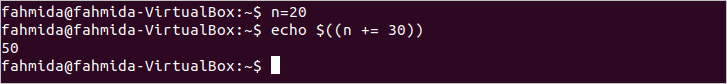Top

### – Integer Operator

‘-‘ is an arithmetic operator that is used to subtraction value of two numbers. The following example shows the use of this operator that will subtract 15 from 35.

\$ echo `expr 35 - 15`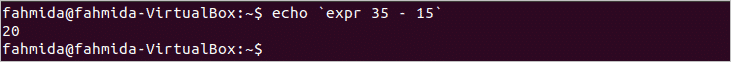Top

### -= Integer Operator

‘-=’ is a shorthand arithmetic operator that subtract numeric value from a variable and store the result in that variable. The following example will subtract 100 from the variable \$n and store the result in \$n.

\$ n=120
\$ echo \$((n -= 100))Top

### * Integer Operator

‘*’ operator is used to multiply number values. The following command shows the use of this operator that will multiply 5 by 7 and print 25 as output.

\$ echo \$((5 * 7))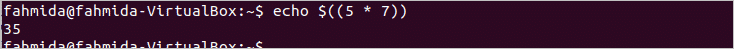Top

### *= Integer Operator

‘*=’ is a shorthand arithmetic operator that multiplies the numeric value with the value of a variable and store that result in that variable. The following command will multiply 50 with the variable \$n and store the result in \$n.

\$ n=10
\$ echo \$((n * 50))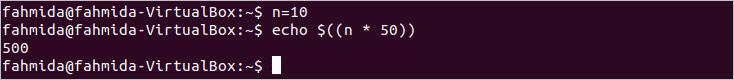Top

### ** Integer Operator

‘**’ operator is used to calculate the xy. ‘**’ is used to print the value of 53 in the following command.

\$ echo \$((5 ** 3))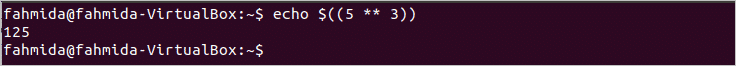Top

### / Integer Operator

‘/’ is an arithmetic operator to divide two numeric values in bash. The following commands show the division of two integer numbers by using `let` command.

\$ let n=30/6
\$ echo \$nTop

### /= Integer Operator

‘/=’ is a shorthand arithmetic operator that divides a variable by a number and store the result into that variable. The following commands will divide \$n by 10 and store the result in \$n.

\$ n=50
\$ let n=n/10
\$ echo \$nTop

### % Integer Operator

‘%’ operator is used to calculate the remainder of the division of two numbers. The remainder value of 89/5 will be printed after executing the following command.

\$ echo `expr 89 % 5`Top

### %= Integer Operator

‘%=’ is a shorthand arithmetic operator that calculates the remainder after dividing the values of a variable by a number and store the remainder value into that variable. The following commands show the use of this operator.

\$ n=150
\$ echo `expr \$n % 7`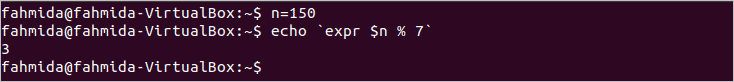Top

### ++ (Pre) Increment Operator

‘++` operator is used to increment the value of a variable by 1. When the operator is used before the variable then it will act as a pre-increment operator that means the value of the variable will be incremented first and will do other operation later. The value of \$i will be incremented before adding with the number 10 in the following example.

\$ i=39
echo \$((++i+10))Top

### (Post) ++ Increment Operator

When ‘++’ operator is used after the variable then it will act as post-increment operator and it increments the value of the variable by 1 after doing another task. In this example, the current value of \$i will be printed first and incremented by 1 in the second command that is 10. The last command will print the value of \$i , which is 11.

\$ i=10
\$ echo \$((i++))
\$ echo \$i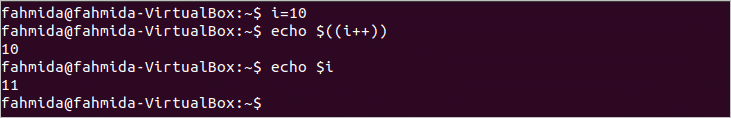Top

### – – (Pre) Decrement Operator

‘–` operator is used to decrement the value of a variable by 1. When the operator is used before the variable then it will act as a pre-decrement operator that means the value of the variable will be decremented first and the other operation will be done later. The value of \$i will be decremented before adding with the number 15 in the following example.

\$ i=36
\$ echo \$((--i+15))Top

### (Post) – – Decrement Operator

If ‘–‘ operator is used after the variable, then it will act as a post-decrement operator and it decrements the value of the variable by 1 after doing another task. In this example, the current value of \$i will be printed first and decremented by 1 in the second command that is 6. The last command will print the value of \$i after decrement, which is 5.

\$ i=6
\$ echo \$((i--))
\$ echo \$iTop

### && Logical Operator

‘&&’ is a comparison operator that is used for creating Boolean AND logic. When all conditions are true the then AND logic return true. Two conditions are checked by using ‘&&’ operator in the following example.

if [[ \$1 = "fahmida" && \$2 = "abcd" ]]
then
echo "Valid user"
else
echo "Invalid user"
fi

The script is executed two times with valid data and invalid data in the following output.Top

### || Logical Operator

‘||’ operator is used to create two or more conditions with OR logic which returns true when any one of the condition returns true. The following script shows the use of this operator.

if [[ \$1 = 101 || \$1 = 780 ]]
then
echo "You have won the ticket"
else
echo "Try again"
fi

The script is executed two times with 101 and 102 in the following output.Top

### ! Logical Operator

‘!’ operator is used to create logical NOT condition that changes true to false or false to true. The following script shows the use of this operator. The script will print “Program is running” if the first command-line argument is empty and print “Program is terminated” if the first command-line argument contains any value.

terminate=\$1
if [[ !\$terminate  ]]
then
echo "Program is running"
else
echo "Program is terminated"
fi

The script is executed without argument and with the argument in the following example.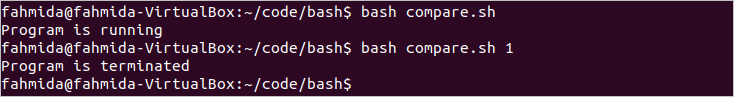Top

### ?: Ternary Operator

‘?:’ operator can be used as an alternative of if statement. The logical condition is defined before ‘?’  and if the condition returns true then it will execute the statement that is defined before ‘:’ otherwise it will execute the statement that is defined after ‘:’. The following script shows the use of this operator.

n=20
v1=100
v2=200
echo \$(( n>=20 ? v1 : v2 ))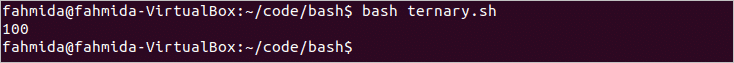Top

### , comma Operator

‘,’ operator is used to execute multiple statements in a line. The following command shows the use of this operator. The value of \$n is assigned to 10, 30 is added with \$n and the value of \$n is printed.

\$ echo "\$(( n=10, n=n+30 ))"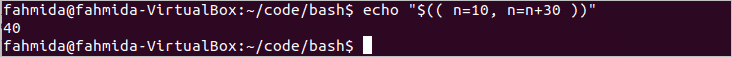Top

### & Bitwise Operator

‘&’ operator is used to perform bitwise AND operation that works on binary data.  The following command shows the use of this operator.

\$ echo \$((3 & 6))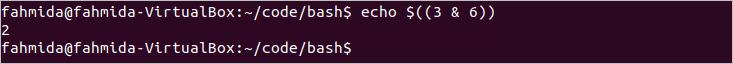Top

### &= Bitwise Operator

‘&=’ operator is used to perform bitwise AND operation with the value of a variable and store the result in the variable. Run the following commands to show the use of this operator.

\$ var=3
\$ ((var&=7))
\$ echo \$varTop

### | Bit-wise Operator

‘|’ operator is used to perform bit-wise OR operation that works on binary data.  The following command shows the use of this operator.

\$ echo \$((3 | 6))Top

### |= Bitwise Operator

‘|=’ operator used is to perform bitwise OR operation with the value of a variable and store the result in the variable. Run the following commands to show the use of this operator.

\$ var=4
\$ ((var|=2))
\$ echo \$varTop

### ^ Bitwise Operator

‘^’ operator is used to perform bitwise XOR operation that works on binary data.  The following command shows the use of this operator.

\$ echo \$((3 ^ 6))Top

### ^= Bitwise Operator

‘^=’ operator is used to perform bitwise XOR operation with the value of a variable and store the result in the variable. Run the following commands to show the use of this operator.

\$ var=5
\$ ((var^=2))
\$ echo \$varTop

### ~ Bitwise Operator

‘~’ operator is used to complement the value. The following command shows the use of this operator. The complement of 7 is -8.

\$ echo \$(( ~7 ))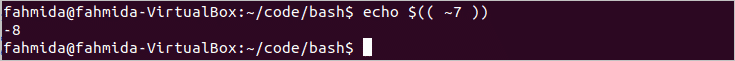Top

### << Bitwise Operator

‘<<‘ operator is used to left-shift the binary value. The following command shows the use of this operator.

\$ echo \$(( 6<<1 ))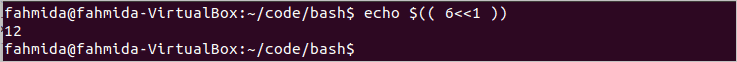Top

### <<= Bitwise Operator

‘<<=’ operator is used to left shift the binary value of any variable and store the value in that variable. The following command shows the use of this operator.

\$ var=5
\$ ((var <<= 1))
\$ echo \$varTop

### >> Bitwise Operator

‘>>’ operator is used to right-shift the binary value. The following command shows the use of this operator.

\$ echo \$(( 8>>1 ))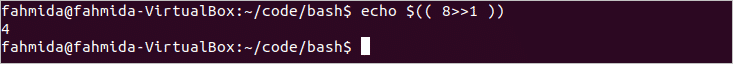Top

### >>= Bitwise Operator

‘>>=’ operator is used to right-shift the binary value of any variable and store the value in that variable. The following command shows the use of this operator.

\$ var=7
\$ ((var >>= 1))
\$ echo \$varTop

### <<< here-string Operator

‘<<<‘ operator is used to passing the data from the right side to standard input. The following command shows the use of this operator.

\$ cat <<< "Linux Hint"Top

### -eq Integer operator

‘-eq’ operator is used to check two values are equal or not. If the values are equal then it returns true otherwise returns false.

n=50
if [ \$n -eq 80 ]
then
echo "The number is equal to 80"
else
echo "The number is not equal to 80"
fi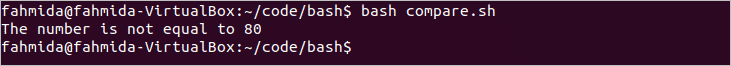Top

### -ne Integer operator

‘-ne’ operator is used to check two numbers are not equal or equal. If the values are not equal then it returns true otherwise returns false.

n=50
if [ \$n -ne 100 ]
then
echo "The number is not equal to 100"
else
echo "The number is equal to 100"
fi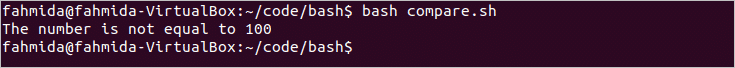Top

### -gt Integer operator

‘-gt’ operator is used to compare two numbers and it returns true if any number is greater than the other number. The following script shows the use of this operator.

n=50
if [ \$n -gt 50 ]
then
echo "The number is greater than 50 "
else
echo "The number is less than or equal to 50"
fi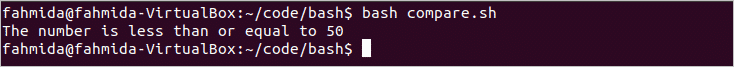Top

### -ge Integer operator

‘-ge’ operator is used to compare two numbers and it returns true if any number is greater than or equal to the other number. The following script shows the use of this operator.

n=50
if [ \$n -ge 50 ]
then
echo "The number is greater than or equal to 50"
else
echo "The number is less than 50"
fi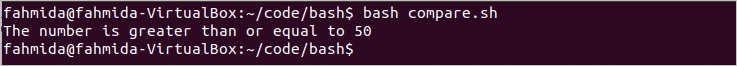Top

### -lt Integer operator

‘-lt’ operator is used to compare two numbers and it returns true if any number is less than the other number. The following script shows the use of this operator.

n=50
if [ \$n -lt 50 ]
then
echo "The number is less than 50"
else
echo "The number is greater than or equal to 50"
fi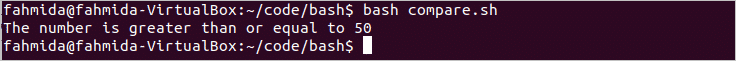Top

### -le Integer operator

‘-le’ operator is used to compare two numbers and it returns true if any number is less than or equal to the other number. The following script shows the use of this operator.

n=50
if [ \$n -le 50 ]
then
echo "The number is less than or equal to 50"
else
echo "The number is greater than 50"
fi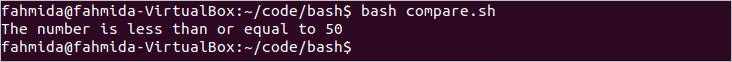Top

### < Integer operator

‘<‘ operator is used to compare two numbers and it returns true if any number is less than the other number. The following script shows the use of this operator.

n=50
if [[ \$n < 50 ]]
then
echo "The number is less than 50"
else
echo "The number is greater than or equal to 50"
fiTop

### <= Integer operator

‘<=’ operator is used to compare two numbers and it returns true if any number is less than or equal to the other number. The following script shows the use of this operator.

n=55
if (( \$n <= 50 ))
then
echo "The number is less than or equal to 50"
else
echo "The number is greater than 50"
fi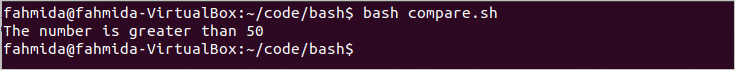Top

### > Integer operator

‘>’ operator is used to compare two numbers and it returns true if any number is greater than the other number. The following script shows the use of this operator.

n=55
if (( \$n > 50 ))
then
echo "The number is greater than 50"
else
echo "The number is less than or equal to 50"
fi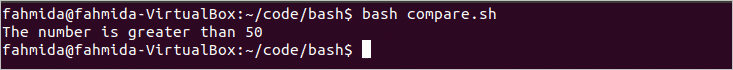Top

### >= Integer operator

‘>=’ operator is used to compare two numbers and it returns true if any number is greater than or equal to the other number. The following script shows the use of this operator.

n=55
if (( \$n >= 55 ))
then
echo "The number is greater than or equal to 55"
else
echo "The number is less than 55"
fiTop

### = String Operator

‘=’ operator is used to compare the equality of two string values. The following script shows the use of this operator.

str="Mango"
if [ \$str = "Orange" ]
then
echo "The value are equal"
else
echo "The value are not equal"
fiTop

### == Equality Operator

‘==’ operator is used to compare the equality of two values. The following script shows the use of this operator.

var=100
if [ \$var == 100 ]
then
echo "The value is equal to 100"
else
echo "The value is not equal to 100"
fiTop

### != Inequality operator

‘!=’ operator is used to comparing the inequality of two values. The following script shows the use of this operator.

var=50
if [ \$var != 100 ]
then
echo "The value is not equal to 100"
else
echo "The value is equal to 100"
fi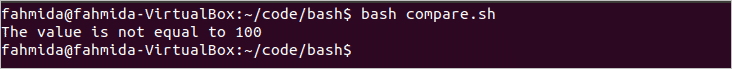Top

### < string operator

‘<‘ operator is used to compare two string values and it returns true if the first value is less than second value. The following script shows the use of this operator.

str1="Mango"
str2="Orange"
if [[ \$str < \$str2 ]]
then
echo "\$str1 is lower than \$str2"
else
echo "\$str1 is greater than \$str2"
fi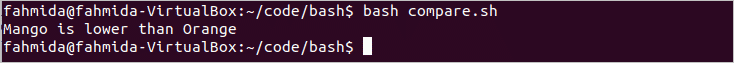Top

### > string operator

‘>’ operator is used to compare two string values and it returns true if the first value is greater than the second value. The following script shows the use of this operator.

str1="Mango"
str2="Orange"
if [[ \$str > \$str2 ]]
then
echo "\$str1 is greater than \$str2"
else
echo "\$str2 is greater than \$str1"
fi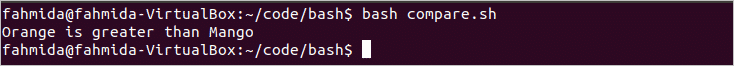Top

### -z string operator

‘-z’ operator is used to check the length of a string is zero or not. The following script shows the use of this operator.

str=""
if [ -z \$str ]
then
echo "The string length is zero"
else
echo "The string length is more than zero"
fi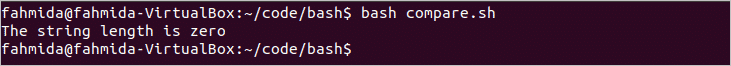Top

### -n string operator

‘-n’ operator is used to check the length of a string is non-zero or not. The following script shows the use of this operator.

str="Linux"
if [ -n \$str ]
then
echo "The string length is non-zero"
else
echo "The string length is zero"
fi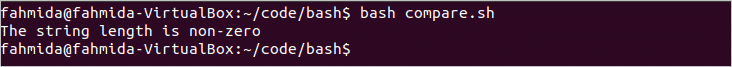Top

### -a logical operator

‘-a’ operator is used to create Boolean AND logic within two or more conditions. The following script shows the use of this operator.

n1=25
n2=65
if [ \$n1 -gt 24 -a \$n2 -lt 66 ]
then
echo "You are eligible"
else
echo "You are not eligible"
fiTop

### -o logical operator

‘-o’ operator is used to create Boolean OR logic within two or more conditions. The following script shows the use of this operator.

score1=55
score2=75
if [ \$score1 -eq 55 -o \$score2 -eq 80 ]
then
echo "You have passed"
else
echo "You have failed"
fi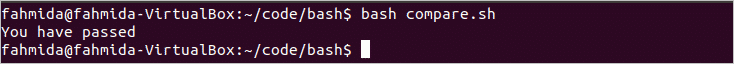Top

### -e file operator

-e test operator is used to check any file or folder is exists or not. Create a bash file with the following script to check any file exists or not. Here, the filename will provide as command-line argument in the script.

filename=\$1
if [ -e \$filename ]
then
echo "File or Folder exists."
else
echo "File or Folder does not exist."
fi

Run the following commands to check the output.

\$ ls
\$ bash fo.sh temp
\$ bash fo.sh test.txt
\$ bash fo.sh testing.txt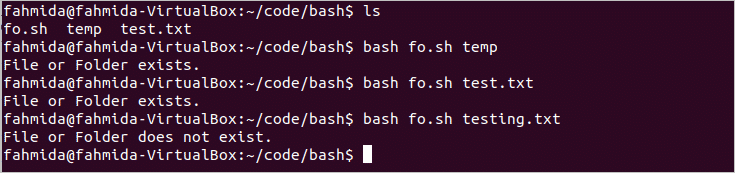Top

### -f file operator

‘-f’ operator is used to check any file exists or not. The following script shows the use of this operator.

if [ -f "test.txt" ]
then
echo "File exists."
else
echo "File does not exist."
fi
\$ ls
\$ bash fo.shTop

### -s file operator

‘-s’ operator is used to check the file size is more than zero or not. The following script shows the use of this operator.

filename=\$1
if [ -s \$filename ]
then
echo "File size is more than zero."
else
echo "File size is zero."
fi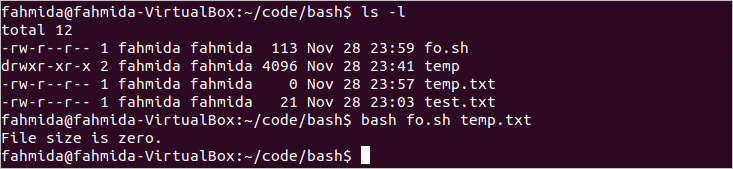Top

### -d file operator

‘-d’ operator is used to check any folder exists or not. The following script shows the use of this operator.

name=\$1
if [ -d \$name ]
then
echo "Folder exists."
else
echo "Folder does not exist."
fi

\$ ls
\$ bash fo.sh temp
\$ bash fo.sh mydir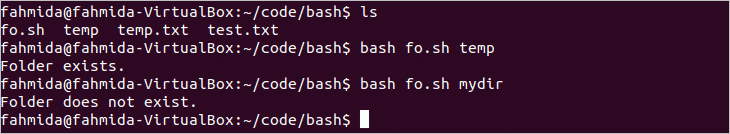Top

### -b file operator

‘-b’ operator is used to check the file is a block special file or not. The following script shows the use of this operator.

name=\$1
if [ -b \$name ]
then
echo "This is a block special file."
else
echo "This is not a block special file."
fi
\$ bash fo.sh /dev/sda1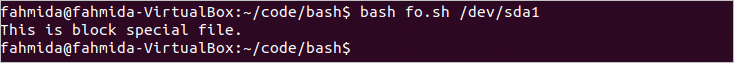Top

### -c file operator

‘-c’ operator is used to check the file is a character special file or not. The following script shows the use of this operator.

name=\$1
if [ -c \$name ]
then
echo "This is a character special file."
else
echo "This is not a character special file."
fi
\$ bash fo.sh /dev/stdin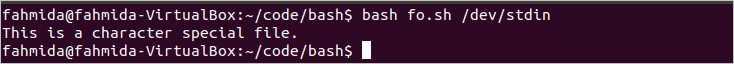Top

### -p file operator

‘-p’ operator is used to check the file is a pipe or not. The following script shows the use of this operator.

pipe_test()
{
[ -p /dev/fd/0 ] && echo "File is a pipe" || echo "File is not a pipe"
}
echo "Hello" | pipe_test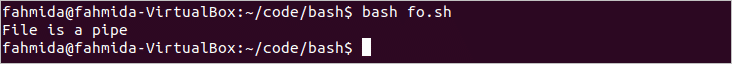Top

### -h file operator

‘-h’ operator is used to check the file is a symbolic link or not. The following script shows the use of this operator.

name=\$1
if [ -h \$name ]
then
echo "It is a symbolic link."
else
echo "It is not a symbolic link."
fi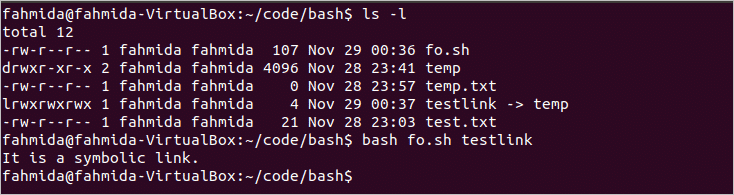Top

### -L file operator

It works like the -h operator mentioned before.

name=\$1
if [ -L \$name ]
then
echo "It is a symbolic link."
else
echo "It is not a symbolic link."
fi

Top

### -S file operator

‘-S’ operator is used to check the file is a socket or not. The following script shows the use of this operator.

name=\$1
if [ -S \$name ]
then
echo "It is a socket."
else
echo "It is not a socket."
fi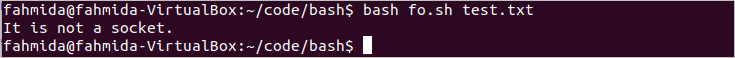Top

### -t file operator

-t’ operator is used to check the file is associated with the terminal or not. The following script shows the use of this operator.

if [ -t 1 ]
then
echo "File is associated with a terminal."
else
echo "File is not associated with the terminal."
fi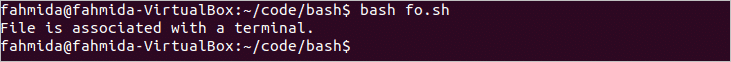Top

### -r file operator

‘-r’ operator is used to check the read permission of a file. The following script shows the use of this operator.

name=\$1
if [ -r \$name ]
then
else
echo "File does not have read permission."
fi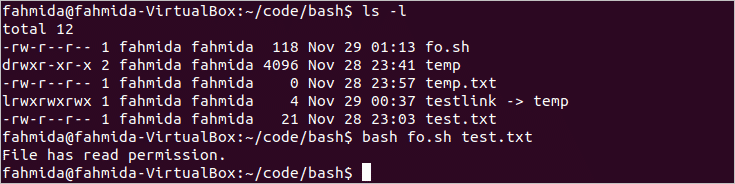Top

### -w file operator

‘-w’ operator is used to check the write permission of a file. The following script shows the use of this operator.

name=\$1
if [ -w \$name ]
then
echo "File has write permission."
else
echo "File does not have write permission."
fi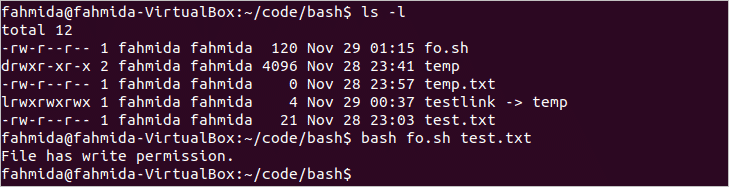Top

### -x file operator

‘-x’ operator is used to check the execution permission of a file. The following script shows the use of this operator.

name=\$1
if [ -x \$name ]
then
echo "File has execution permission."
else
echo "File does not have execution permission."
fi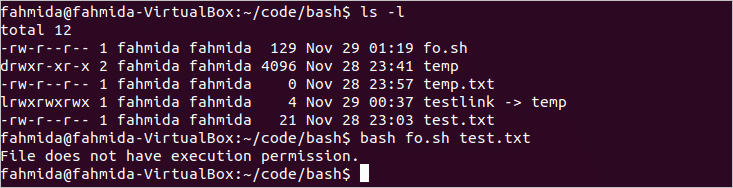Top

### -g file operator

‘-g’ operator is used to check the group id (SGID) is set or not for a file. The following script shows the use of this operator.

name=\$1
if [ -g \$name ]
then
echo "Group id is set."
else
echo "Group id is not set."
fiTop

### -u file operator

‘-u’ operator is used to check the user id (SUID) is set or not for a file. The following script shows the use of this operator.

if [ -u \$1 ]
then
echo "User id is set."
else
echo "User id is not set."
fi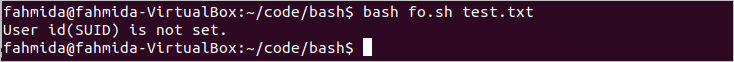Top

### -k file operator

‘-k’ operator is used to check the sticky bit is set or not for a file. The following script shows the use of this operator.

if [ -k \$1 ]
then
echo "Sticky bit is set."
else
echo "Sticky bit is not set."
fi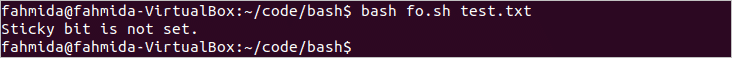Top

### -O file operator

‘-O’ operator is used to check the ownership of the file. The following script shows the use of this operator.

if [ -O \$1 ]
then
echo "Owner of the file."
else
echo "Not the owner of the file."
fi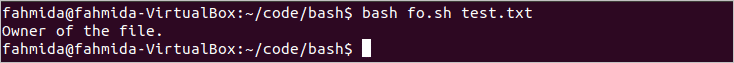Top

### -G file operator

‘-G’ operator is used to check both group id of the file and the login user is the same. The following script shows the use of this operator.

if [ -G \$1 ]
then
echo "Group Id are the same."
else
echo "Group Id are not the same."
fiTop

### -N file operator

‘-N’ operator is used to check any file is modified or not. The following script shows the use of this operator.

if [ -N \$1 ]
then
echo "File is modified."
else
echo "File is not modified."
fi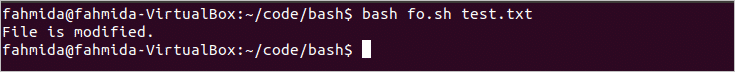Top

### -nt file operator

‘-nt’ operator is used to check that any file is newer than the other file or not. The following script shows the use of this operator.

if [ \$1 -nt \$2 ]
then
echo "\$1 is newer than \$2"
else
echo "\$2 is newer than \$1"
fi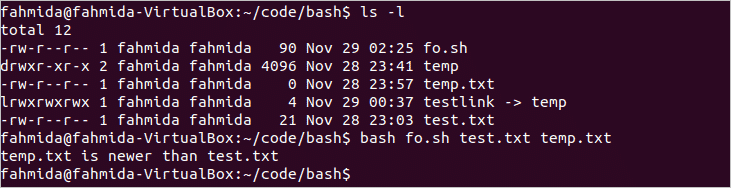Top

### -ot file operator

‘-ot’ operator is used to check any file is older than the other file or not. The following script shows the use of this operator.

if [ \$1 -ot \$2 ]
then
echo "\$1 is older than \$2"
else
echo "\$2 is older than \$1"
fi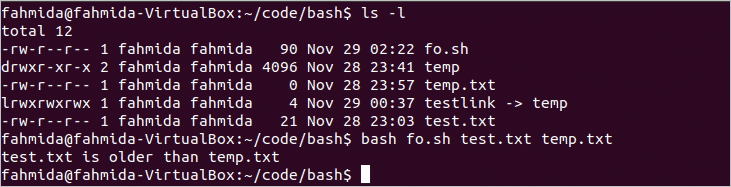Top

### -ef file operator

‘-ef’ operator is used to check that two hard links are pointing the same file or not. The following example shows the use of this operator.

if [ \$1 -ef \$2 ]
then
echo "\$1 and \$2 are hard links of the same file."
else
echo "\$1 and \$2 are not hard links of the same file."
fi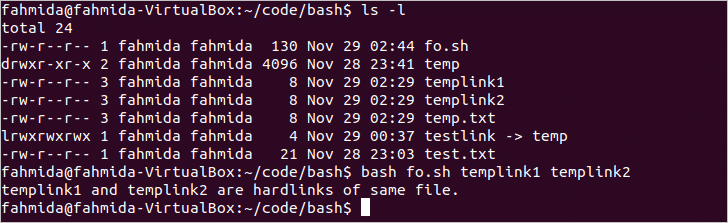#### Conclusion

The most common uses of bash operators are explained in this article with very simple examples. It will help the new bash programmer to use bash operators for various purposes.